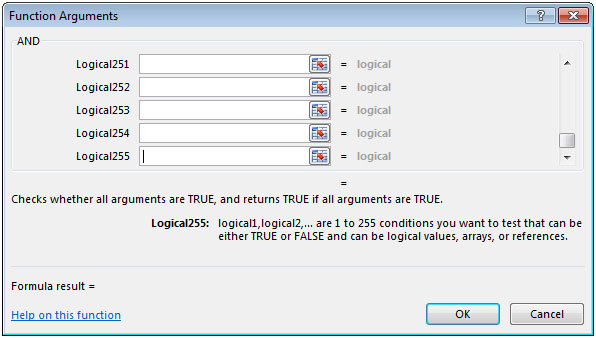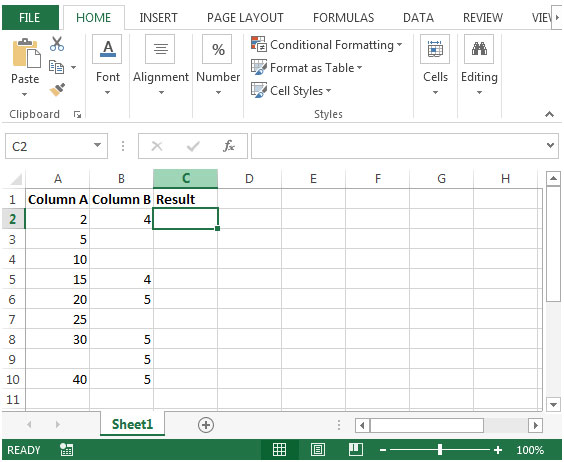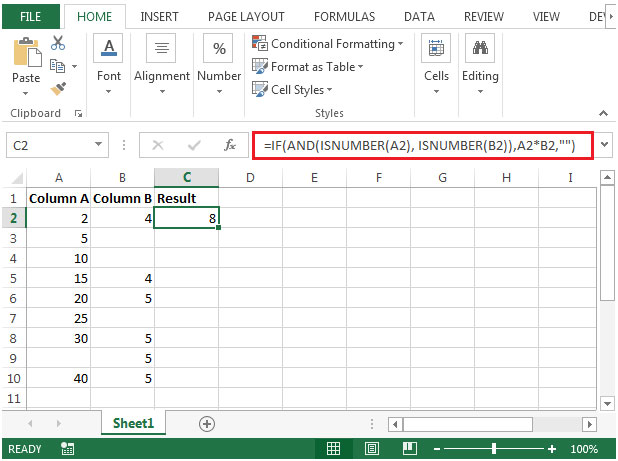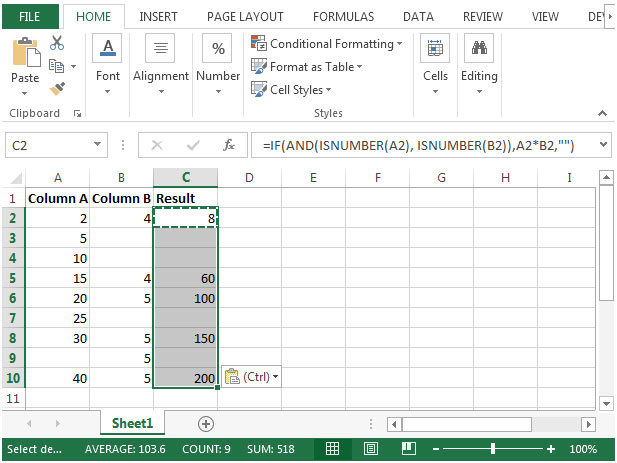# Ignoring Blank Cells When Performing Calculations In Microsoft Excel 2010

In this article, we will learn ignoring blank cells when performing calculations in Microsoft Excel 2010.

Consider a scenario in which you have two columns that contain numbers & empty cells. In the third column, you want to multiply the value in one column to another by ignoring blank cells while performing calculations & the formula should not give any false results or errors.

You can use a combination of IF, AND & ISNUMBER functions to get the output.

IF function checks whether the condition you specify is TRUE or FALSE. If the condition returns TRUE then it returns preset value, and if the condition returns FALSE, then it returns another preset value.

Syntax = IF(logical_test,value_if_true,value_if_false)

logical_test: Logical test will test the condition or criteria. If the condition meets then it returns the preset value, and if the condition does not meet then it returns another preset value.

value_if_true: The value that you want to be returned if this argument returns TRUE.

value_if_false: The value that you want to be returned if this argument returns FALSE

AND: Checks whether all the arguments are TRUE, and returns TRUE if all the arguments are TRUE. If anyone is found to be FALSE, then AND function will returns FALSE.

Syntax =AND(logical1,logical2,...)

logical1: The first condition that you want to check

logical2: This is optional. You can add as many as 255 conditions at a time to evaluate TRUE or FALSE.ISNUMBER: Checks whether a value is a number, and returns TRUE or FALSE.

Syntax =ISNUMBER(value)

value: It is the value that you want to check whether it is a number or not.

Let us take an example:

• We have some numbers in column A & B. There can be empty cell in either of the columns.
• In column C, a formula is required to derive the output as by multiplying the value from one column to another, provided there should be no false results or errors.

Say if we multiply value of 5 with empty cells then the result will 0, which is a false result.• In cell C2, the formula would be

=IF(AND(ISNUMBER(A2), ISNUMBER(B2)),A2*B2,"")• AND function checks two conditions at a time i.e. if the numbers in the column A & B are numbers or not.
• If value in both the columns is a number then both the conditions will meet. AND function will return TRUE.
• IF function will check the value passed by AND function as TRUE, and then multiply values from both the columns, and will return the output in column C.
• If AND function has found any one condition not meeting the criteria, then IF function will return the output as blank (“”).
• Copying the formula from cell C2 to range C3:C10, we will get the desired output.Terms and Conditions of use

The applications/code on this site are distributed as is and without warranties or liability. In no event shall the owner of the copyrights, or the authors of the applications/code be liable for any loss of profit, any problems or any damage resulting from the use or evaluation of the applications/code.